# Pattern in the column

Hello:-)

Let’s say I have a column of 1000 numbers. In the column I have random numbers and I would like to find numbers which can be found in a series of 5.
For example:
2, 5, 24, 3, 69, 37, 1, 45, 62, 65, 90, 13, 17,14, 4, 9… and I would like to end up with: 5, 45, 65, 90 (one serie) and 4, 9, 14, 24 (the second serie).

Is there an easy way how obtain desire results?Amanda

Hi @Amanda252 , this is easily achieved using the mod() function from the Math Formula:

You can do:
`mod(\$your column\$, 5)`

This will give you the remainder when dividing the values from your column by 5.

For example:

``````mod(10, 5) = 0
mod(1, 5) = 1
mod(24, 5) = 4
mod(69, 5) = 4
``````

etc

You can then filter on this result and filter by 0 and 4.

Those with 0 will be the series for multiples of 5, and will give you numbers like 5, 45, 65, 90.

Those with 4 will be the series with remainder of 4 when divided by 5, and will give you numbers like 4, 9, 14, 24.

3 Likes

Hi @Amanda252 , here’s something I put together for you: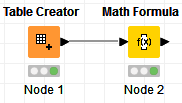Input: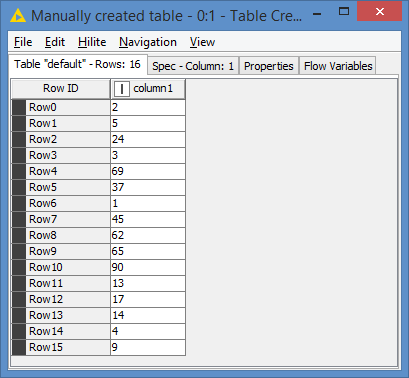Math Formula:

Expression: `mod(\$column1\$, 5)`

Results: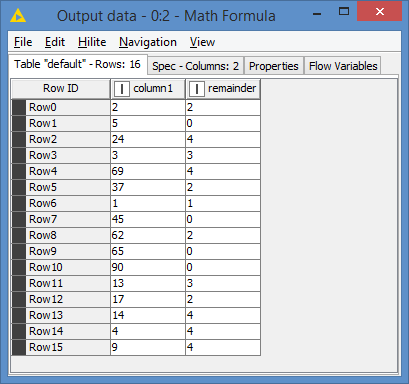From there, you can filter out anything that’s not 0 or 4:

I’m using the Rule-based Row Filter as you can see, and the expression is:
`\$remainder\$ IN (0, 4) => TRUE`

Results:I can now split between 0 and 4 using the Row Splitter:

Final workflow looks like this: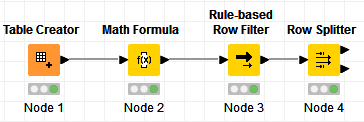Top Series: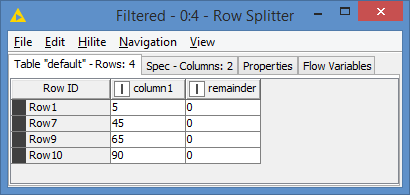Bottom Series: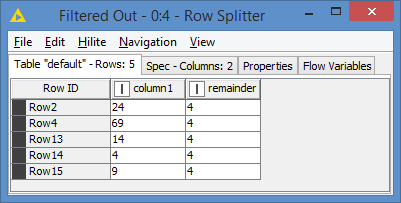2 Likes

Thank you very much for your help. It works perfectly. Thanks

3 Likes